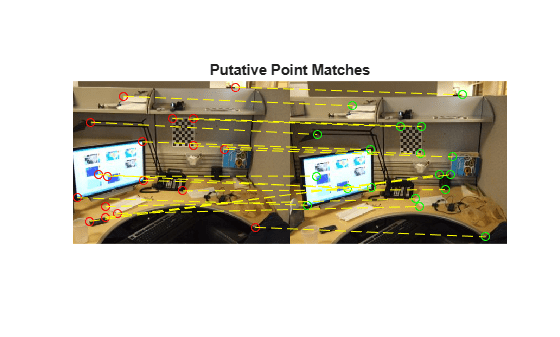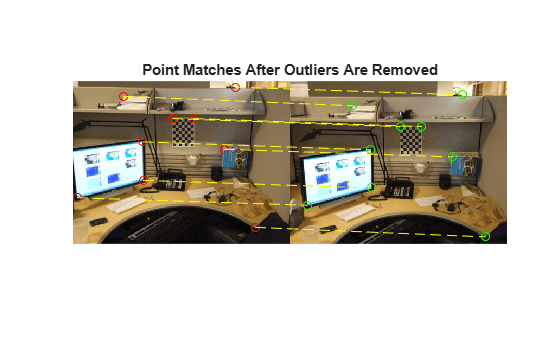# estimateFundamentalMatrix

Estimate fundamental matrix from corresponding points in stereo images

## Syntax

``estimateFundamentalMatrix``
``F = estimateFundamentalMatrix(matchedPoints1,matchedPoints2)``
``````[F,inliersIndex] = estimateFundamentalMatrix(matchedPoints1,matchedPoints2)``````
``````[F,inliersIndex,status] = estimateFundamentalMatrix(matchedPoints1,matchedPoints2)``````
``````[F,inliersIndex,status] = estimateFundamentalMatrix(matchedPoints1,matchedPoints2,Name=Value)``````

## Description

example

````estimateFundamentalMatrix` estimates the fundamental matrix from corresponding points in stereo images. This function can be configured to use all corresponding points or to exclude outliers. You can exclude outliers by using a robust estimation technique such as random-sample consensus (RANSAC). When you use robust estimation, results may not be identical between runs because of the randomized nature of the algorithm.```

example

````F = estimateFundamentalMatrix(matchedPoints1,matchedPoints2)` returns the 3-by-3 fundamental matrix, `F`, using the least median of squares (LMedS) method from matched feature points in stereo images.```
``````[F,inliersIndex] = estimateFundamentalMatrix(matchedPoints1,matchedPoints2)``` additionally returns logical indices, `inliersIndex`, for the inliers used to compute the fundamental matrix. The `inliersIndex` output is an M-by-1 vector. The function sets the elements of the vector to `true` when the corresponding point was used to compute the fundamental matrix. The elements are set to `false` if they are not used.```
``````[F,inliersIndex,status] = estimateFundamentalMatrix(matchedPoints1,matchedPoints2)``` additionally returns a status code.```
``````[F,inliersIndex,status] = estimateFundamentalMatrix(matchedPoints1,matchedPoints2,Name=Value)``` specifies options using one or more name-value arguments in addition to any combination of arguments from previous syntaxes. For example, `estimateFundamentalMatrix(matchedPoints1,matchedPoints2,Method="MSAC")` specifies MSAC as the method to compute the fundamental matrix.```

## Examples

collapse all

Use the random sample consensus (RANSAC) method to compute the fundamental matrix. The RANSAC method requires that the input points are putatively matched. You can use the matchFeatures function to return these matched points. Outlier points which may still be contained within putatively matched points are further eliminated by using the RANSAC algorithm.

Load stereo points into the workspace.

`load stereoPointPairs`

Estimate the fundamental matrix.

```fRANSAC = estimateFundamentalMatrix(matchedPoints1, ... matchedPoints2,Method="RANSAC", ... NumTrials=2000,DistanceThreshold=1e-4)```
```fRANSAC = 3×3 0.0000 -0.0004 0.0348 0.0004 0.0000 -0.0937 -0.0426 0.0993 0.9892 ```

Load the putatively matched points into the workspace.

```load stereoPointPairs [fLMedS,inliers] = estimateFundamentalMatrix(matchedPoints1,matchedPoints2,NumTrials=2000)```
```fLMedS = 3×3 0.0000 -0.0004 0.0349 0.0004 0.0000 -0.0938 -0.0426 0.0994 0.9892 ```
```inliers = 18x1 logical array 1 1 1 1 1 1 0 1 0 0 ⋮ ```

```I1 = imread("viprectification_deskLeft.png"); I2 = imread("viprectification_deskRight.png");```

Show the putatively matched points.

```figure; showMatchedFeatures(I1,I2,matchedPoints1,matchedPoints2,"montage",PlotOptions=["ro","go","y--"]); title("Putative Point Matches");```Show the inlier points.

```figure; showMatchedFeatures(I1,I2,matchedPoints1(inliers,:),matchedPoints2(inliers,:),"montage",PlotOptions=["ro","go","y--"]); title("Point Matches After Outliers Are Removed");```Load the stereo point pairs into the workspace.

`load stereoPointPairs`

Compute the fundamental matrix for input points which do not contain any outliers.

```inlierPts1 = matchedPoints1(knownInliers,:); inlierPts2 = matchedPoints2(knownInliers,:); fNorm8Point = estimateFundamentalMatrix(inlierPts1,inlierPts2,Method="Norm8Point")```
```fNorm8Point = 3×3 0.0000 -0.0004 0.0348 0.0004 0.0000 -0.0937 -0.0426 0.0993 0.9892 ```

## Input Arguments

collapse all

Coordinates of corresponding points in image one, specified as an M-by-2 matrix of M number of [x y] coordinates, or as one of the point feature objects described in Point Feature Types. The `matchedPoints1` input must contain points which do not lie on a single planar surface, (e.g., a wall, table, or book) and are putatively matched by using a function such as `matchFeatures`.

Coordinates of corresponding points in image one, specified as an M-by-2 matrix of M number of [x y] coordinates, or as one of the point feature objects described in Point Feature Types. The `matchedPoints1` input must contain points which do not lie on a single planar surface, (e.g., a wall, table, or book) and are putatively matched by using a function such as `matchFeatures`.

### Name-Value Arguments

Specify optional pairs of arguments as `Name1=Value1,...,NameN=ValueN`, where `Name` is the argument name and `Value` is the corresponding value. Name-value arguments must appear after other arguments, but the order of the pairs does not matter.

Before R2021a, use commas to separate each name and value, and enclose `Name` in quotes.

Example: `estimateFundamentalMatrix(matchedPoints1,matchedPoints2,Method="MSAC")` specifies MSAC as the method to compute the fundamental matrix.

Method used to compute the fundamental matrix, specified as one of the following:

 `"LMedS"` Least Median of Squares. Use this method if at least 50% of the points in `matchedPoints1` and `matchedPoints2` are inliers. The function stops the search for inliers and the estimation of the fundamental matrix after 50% of the inliers are found. `"MSAC"` M-estimator SAmple Consensus. Select the M-estimator SAmple Consensus method if you would like to set the distance threshold for the inliers. Generally, the MSAC method converges more quickly than the RANSAC method. `"Norm8Point"` Normalized eight-point algorithm  . To produce reliable results, the inputs, `matchedPoints1` and `matchedPoints2` must match precisely.

To produce reliable results using the `Norm8Point` algorithm, the inputs, `matchedPoints1` and `matchedPoints2`, must match precisely. The other methods can tolerate outliers and therefore only require putatively matched input points. You can obtain putatively matched points by using the `matchFeatures` function.

Fundamental matrix class, specified as `"double"` or `"single"`. Use this property to specify the class for the fundamental matrix and for the function's internal computations.

Number of random trials for finding the outliers, specified as an integer. This argument applies when you set the `Method` to `LMedS` or `MSAC`.

• `LMedS` — The function uses the actual number of trials as the parameter value.

• `MSAC` — The function uses the maximum number of trials as the parameter value.

The actual number of trials depends on `matchedPoints1`, `matchedPoints2`, and the value of the `Confidence` parameter. Select the number of random trials to optimize speed and accuracy.

Distance threshold for finding outliers, specified as a positive scalar. This parameter applies when you set the `Method` to `MSAC`.

Desired confidence for finding maximum number of inliers, specified as a percentage scalar in the range `(0 100)`. This argument applies when you set `Method` to `MSAC`.

Report runtime error, specified as a logical value. Set this parameter to `true` to report run-time errors when the function cannot compute the fundamental matrix from `matchedPoints1` and `matchedPoints2`. When you set this parameter to `false`, you can check the `status` output to verify validity of the fundamental matrix.

## Output Arguments

collapse all

Fundamental matrix, returned as a 3-by-3 matrix that is computed from the points in the inputs `matchedPoints1` and `matchedPoints2`.

`$\left[\begin{array}{cc}{P}_{2}& 1\end{array}\right]*FundamentalMatrix*{\left[\begin{array}{cc}{P}_{1}& 1\end{array}\right]}^{\text{'}}=0$`

P1, the point in `matchedPoints1` of image 1 in pixels, corresponds to the point, P2, the point in `matchedPoints2` in image 2.

In computer vision, the fundamental matrix is a 3-by-3 matrix which relates corresponding points in stereo images. When two cameras view a 3-D scene from two distinct positions, there are a number of geometric relations between the 3-D points and their projections onto the 2-D images that lead to constraints between the image points. Two images of the same scene are related by epipolar geometry.

Inliers index, returned as an M-by-1 logical index vector. An element set to `true` means that the corresponding indexed matched points in `matchedPoints1` and `matchedPoints2` were used to compute the fundamental matrix. An element set to `false` means the indexed points were not used for the computation.

Data Types: `logical`

Status code, returned as one of the following possible values:

statusValue
`0`:No error.
`1`:`matchedPoints1` and `matchedPoints2` do not contain enough points. The `Norm8Point` and `MSAC` methods require at least 8 points, and the `LMedS` method requires 16 points.
`2`:Not enough inliers found.

Data Types: `int32`

## Tips

Use `estimateEssentialMatrix` when you know the camera intrinsics. You can obtain the intrinsics using the Camera Calibrator app. Otherwise, you can use the `estimateFundamentalMatrix` function that does not require camera intrinsics. Note that the fundamental matrix cannot be estimated from coplanar world points.

## Algorithms

collapse all

### Computing the Fundamental Matrix

When you choose the `Norm8Point` method, the function uses all points in `matchedPoints1` and `matchedPoints2` to compute the fundamental matrix. When you choose any other method, the function uses the following algorithm to exclude outliers and compute the fundamental matrix from inliers:

1. Initialize the fundamental matrix, F, to a 3-by-3 matrix of zeros.

2. Set the loop counter n, to zero, and the number of loops N, to the number of random trials specified.

3. Loop through the following steps while n < N:

1. Randomly select 8 pairs of points from `matchedPoints1` and `matchedPoints2`.

2. Use the selected 8 points to compute a fundamental matrix, f, by using the normalized 8-point algorithm.

3. Compute the fitness of f for all points in `matchedPoints1` and `matchedPoints2`.

4. If the fitness of f is better than F, replace F with f.

For the `MSAC` method, update N.

5. n = n + 1

### Number of Random Samplings for the MSAC Method

The MSAC method updates the number of random trials N for every iteration in the algorithm loop. The function resets N, according to the following:

 N = min( N, $\frac{\mathrm{log}\left(1-p\right)}{\mathrm{log}\left(1-{r}^{8}\right)}$). Where, p represents the confidence parameter you specified, and r is calculated as follows: $\sum _{i}^{N}\mathrm{sgn}\left(d{u}_{i,}{v}_{i}\right),t\right)/N$, where $\mathrm{sgn}\left(a,b\right)=1$ if $a\le b$ and $0$ otherwise.
When you use the `MSAC` method, results may not be identical between runs because of the randomized nature of the algorithm.

### Sampson Distance

The function uses the Sampson distance to measure the distance of a pair of points according to a fundamental matrix. The equation below can be used to calculate the Sampson distance. In the equation, u represents `matchedPoints1` and v represents `matchedPoints2`.

$d\left({u}_{i},{v}_{i}\right)={\left({v}_{i}F{u}_{i}^{T}\right)}^{2}\left[\frac{1}{{\left(F{u}_{i}^{T}\right)}_{1}^{2}+{\left(F{u}_{i}^{T}\right)}_{2}^{2}}+\frac{1}{{\left({v}_{i}F\right)}_{1}^{2}+{\left({v}_{i}F\right)}_{2}^{2}}\right]$

where $i$ represents the index of the corresponding points, and ${\left(F{u}_{i}^{T}\right)}_{j}^{2}$, the square of the j-th entry of the vector $F{u}_{i}^{T}$.

### Fitness of Fundamental Matrix for Corresponding Points

The following table summarizes how each method determines the fitness of the computed fundamental matrix:

MethodMeasure of Fitness
LMedS$median\left(d\left({u}_{i,}{v}_{i}\right);i=1:N\right)$, the number of input points. The smaller the value, the better the fitness.
MSAC$\sum _{i}^{N}\mathrm{min}\left(d\left({u}_{i},{v}_{i}\right),t\right)$. The smaller the value, the better the fitness.

 Hartley, R., A. Zisserman, Multiple View Geometry in Computer Vision, Cambridge University Press, 2003.

 Rousseeuw, P., A. Leroy, Robust Regression and Outlier Detection, John Wiley & Sons, 1987.

 Torr, P. H. S., and A. Zisserman, MLESAC: A New Robust Estimator with Application to Estimating Image Geometry, Computer Vision and Image Understanding, 2000.